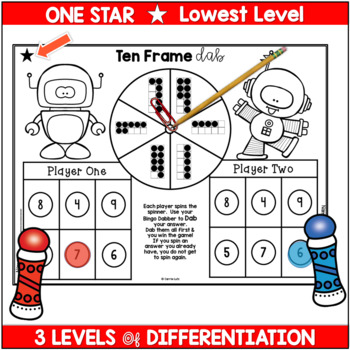DID YOU KNOW:
Seamlessly assign resources as digital activities

Learn how in 5 minutes with a tutorial resource. Try it Now

Learn More# Bingo Dabber Math Centers WorksheetsK - 2nd, Homeschool
Subjects
Standards
Resource Type
Formats Included
• PDF
Pages
30 pages

### Description

Don't you love Math Centers that are differentiated and fun, but require little preparation from you! These Bingo Dauber/Dabber Math Printables are easy, fast and fun. You'll want to grab these for this low price! Your kids will love the cute robot theme!

There are 10 Skills Covered in this IRRESISTIBLE resource:

Ten Frame Dab – Counting Ten Frames, Doubles & Ten Frames plus more

Base Ten Dab – Counting Base Ten Blocks to 20, Base Ten Blocks to 99,

and Base Ten Blocks to 150

More & Less Dab – Ten More/Ten Less, More Than/Less Than, & Odd

and Even More Than/Less Than

Finding Differences Dab – Subtract from 8, Subtract 10, subtract from 18

Money Dab – Count coins to 30 cents no quarters, 41 cents, & 65 cents

Telling Time Dab – Time to the Hour, Time to the Half Hour & Time to Quarter Hours

2 – D Shapes Dab – Name 2-D Shapes, identify shape attributes, & apply shape attributes

3 – D Shapes Dab – Identify 3–D shapes, Recognize shapes in the real world, Identify shape attributes

Fractions – Whole Fractions to 4ths, Whole Fractions to 8ths, Parts of a set to 8ths

There are 3 levels of differentiation for each skill. 1, 2 and 3 Stars are used to show the levels.

My students love using Bingo Dabbers...... They are so engaging!

If I put these activities out at indoor recess, they will play them instead of other games that are provided!

^^^^^^^^^^^^^^^^^^^^^^^^^^^^^^^^^^^^^^^^^^^^^^^^^^^^^^^^^^^^^^^^^^^^^^^^^^^^^^^^^^^^^^^^^^^^^^^^^^^^^^^^^^^^^^^^^^^^^^^^^^^^^^

More BINGO DABBER FUN:

More to LOVE:

Total Pages
30 pages
N/A
Teaching Duration
N/A
Report this Resource to TpT
Reported resources will be reviewed by our team. Report this resource to let us know if this resource violates TpT’s content guidelines.

### Standards

to see state-specific standards (only available in the US).
Recognize and draw shapes having specified attributes, such as a given number of angles or a given number of equal faces. Identify triangles, quadrilaterals, pentagons, hexagons, and cubes.
Compose two-dimensional shapes (rectangles, squares, trapezoids, triangles, half-circles, and quarter-circles) or three-dimensional shapes (cubes, right rectangular prisms, right circular cones, and right circular cylinders) to create a composite shape, and compose new shapes from the composite shape.
Distinguish between defining attributes (e.g., triangles are closed and three-sided) versus non-defining attributes (e.g., color, orientation, overall size); build and draw shapes to possess defining attributes.
Understand the meaning of the equal sign, and determine if equations involving addition and subtraction are true or false. For example, which of the following equations are true and which are false? 6 = 6, 7 = 8 - 1, 5 + 2 = 2 + 5, 4 + 1 = 5 + 2.
Add and subtract within 20, demonstrating fluency for addition and subtraction within 10. Use strategies such as counting on; making ten (e.g., 8 + 6 = 8 + 2 + 4 = 10 + 4 = 14); decomposing a number leading to a ten (e.g., 13 - 4 = 13 - 3 - 1 = 10 - 1 = 9); using the relationship between addition and subtraction (e.g., knowing that 8 + 4 = 12, one knows 12 - 8 = 4); and creating equivalent but easier or known sums (e.g., adding 6 + 7 by creating the known equivalent 6 + 6 + 1 = 12 + 1 = 13).